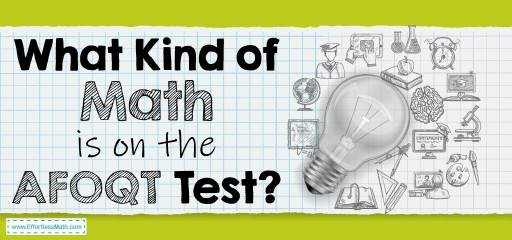# What Kind of Math Is on the AFOQT Test?The AFOQT is a standardized test designed specifically to assess the qualifications and skills of individuals seeking entry into the United States Air Force. After passing this test, a qualified person can be selected to perform the initial stages of training.

AFOQT is a multiple-choice test that includes 12 subtests. In total, test-takers in these 12 subtests must answer 470 questions in 213 minutes (approximately 3.5 hours). This may seem like a long time, but it will pass quickly for you. The two AFOQT subtests are Arithmetic Reasoning and Mathematics Knowledge, and here we briefly review the contents of these two subtests.

## Arithmetic Reasoning

This section tests your ability to use basic arithmetic to solve a variety of math problems, including word problems. It is necessary to study topics such as geometry, proportions, ratios, mixtures, integers, percentages, and time-distance in this section. You must answer 25 questions of arithmetic within 29 minutes, which means you will have a little more than a minute to solve each question. Each question may have up to five possible answers. You must first understand the question well, then you can decide which of the answer options is correct. Usually, the calculations in this section are not difficult and just cover the basics. But the main challenge is to choose which types of calculations need to be performed. The use of calculators is not allowed in this section.

## Mathematics Knowledge

This subtest consists of 25 questions and you have 22 minutes to answer these questions. To complete this section on time, you must be able to solve 1 to 2 questions per minute. In this section, you will encounter straightforward math concepts. Of course, the knowledge you need to answer Mathematics Knowledge questions is more advanced than what is needed to perform calculations for Arithmetic Reasoning problems. The questions in this section focus on the basics of mathematics, including algebra, geometry, and arithmetic. To answer the questions in this section, you must have sufficient knowledge in the field of algebra and geometry. There are many mathematical concepts that you may encounter in the Mathematics Knowledge section. More common concepts include systems of equations, imaginary numbers, ratios, factoring of polynomials, orders of operations, exponents, radicals, functions, probabilities.

## FAQs:

### Is the AFOQT Math test hard?

The AFOQT Math test is not very difficult, but the point that makes this test challenging is that it somehow measures your IQ.

### How do I study for the AFOQT Math?

How you prepare for the AFOQT Math test is very important. You can use good prep books to prepare well for the AFOQT Math test. You can also use online practice tests for further practice after studying the AFOQT exam courses.

### How is the AFOQT Test Scored?

A score of ten is required for officer qualification. for more information, you can check here.

## More from Effortless Math for AFOQT Test …

### Looking for a collection of free math worksheets that would help you in your AFOQT Math preparation?

Check out our AFOQT Math Worksheets: FREE & Printable.

### Want to review the most common AFOQT Math formulas?

Here is our complete list of AFOQT Math formulas.

Have a look at our Full-Length AFOQT Math Practice Test and Free AFOQT Math Practice Test.

## Have any questions about the AFOQT Test?

### What people say about "What Kind of Math Is on the AFOQT Test? - Effortless Math: We Help Students Learn to LOVE Mathematics"?

No one replied yet.

X
52% OFF

Limited time only!

Save Over 52%

SAVE \$40

It was \$76.99 now it is \$36.99